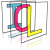Image Component Library (ICL)
icl::math::KMeans< Vector, Scalar > Class Template Reference

Generic Implementation of the K-Means algorithm. More...

`#include <KMeans.h>`

## Classes

struct  Result
restult type More...

## Public Member Functions

KMeans (int numCenters=0)
constructor with optionally given number of centers More...

void init (int numCenters)
deferred intitialization with number of centers More...

int findNN (const Vector &v, Scalar &minDist)
finds the nearest centroid for a given data pointer More...

template<class RandomAcessIterator >
Result apply (RandomAcessIterator begin, RandomAcessIterator end, int numSteps=1000, bool reinitCenters=true)
runs the KMeans algorithms on the given data More...

## Static Private Member Functions

static Scalar diff_power_two (const Scalar &a, const Scalar &b)
internal utility function More...

static Scalar dist (const Vector &a, const Vector &b)
utility function computing the vector distance More...

static void setVectorNull (Vector &v)
sets a vector to null More...

## Private Attributes

std::vector< Vector > m_centers
internal list of centroids More...

std::vector< Vector > m_means
internal buffer More...

std::vector< int > m_nums
numbers of points associated to each centroid More...

std::vector< Scalar > m_errors
averate quantisation error for each centroid More...

## Detailed Description

### template<class Vector, class Scalar> class icl::math::KMeans< Vector, Scalar >

Generic Implementation of the K-Means algorithm.

The K-Means algorithms performs vector quantisation in a very simple way. Given a set of data pointers xi, and starting with a fixed given number of initial centroids, randomly drawn from the given data points, it iterates two steps:

1. assign each data point to it's closest centroid (winnter takes all association)
2. move centroids to the mean of it's associated data points

# Vector Types

Supported Vector Types are FixedColVector, FixedRowVector, DynColVector, DynRowVector and Point32f

## ◆ KMeans()

template<class Vector , class Scalar >
 icl::math::KMeans< Vector, Scalar >::KMeans ( int numCenters = `0` )
inline

constructor with optionally given number of centers

## ◆ apply()

template<class Vector , class Scalar >
template<class RandomAcessIterator >
 Result icl::math::KMeans< Vector, Scalar >::apply ( RandomAcessIterator begin, RandomAcessIterator end, int numSteps = `1000`, bool reinitCenters = `true` )
inline

runs the KMeans algorithms on the given data

if reinitCenters is set to false, the centers of the last call to this method are reused

## ◆ diff_power_two()

template<class Vector , class Scalar >
 static Scalar icl::math::KMeans< Vector, Scalar >::diff_power_two ( const Scalar & a, const Scalar & b )
inlinestaticprivate

internal utility function

## ◆ dist()

template<class Vector , class Scalar >
 static Scalar icl::math::KMeans< Vector, Scalar >::dist ( const Vector & a, const Vector & b )
inlinestaticprivate

utility function computing the vector distance

## ◆ findNN()

template<class Vector , class Scalar >
 int icl::math::KMeans< Vector, Scalar >::findNN ( const Vector & v, Scalar & minDist )
inline

finds the nearest centroid for a given data pointer

## ◆ init()

template<class Vector , class Scalar >
 void icl::math::KMeans< Vector, Scalar >::init ( int numCenters )
inline

deferred intitialization with number of centers

## ◆ setVectorNull()

template<class Vector , class Scalar >
 static void icl::math::KMeans< Vector, Scalar >::setVectorNull ( Vector & v )
inlinestaticprivate

sets a vector to null

## ◆ m_centers

template<class Vector , class Scalar >
 std::vector icl::math::KMeans< Vector, Scalar >::m_centers
private

internal list of centroids

## ◆ m_errors

template<class Vector , class Scalar >
 std::vector icl::math::KMeans< Vector, Scalar >::m_errors
private

averate quantisation error for each centroid

## ◆ m_means

template<class Vector , class Scalar >
 std::vector icl::math::KMeans< Vector, Scalar >::m_means
private

internal buffer

## ◆ m_nums

template<class Vector , class Scalar >
 std::vector icl::math::KMeans< Vector, Scalar >::m_nums
private

numbers of points associated to each centroid

The documentation for this class was generated from the following file:
• /Users/alneuman/vm/icl/ICLMath/src/ICLMath/KMeans.h